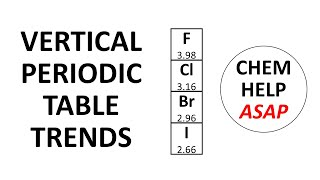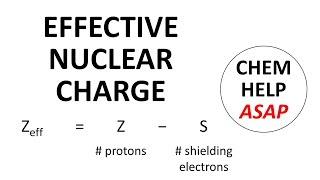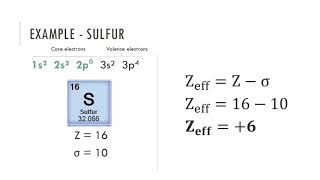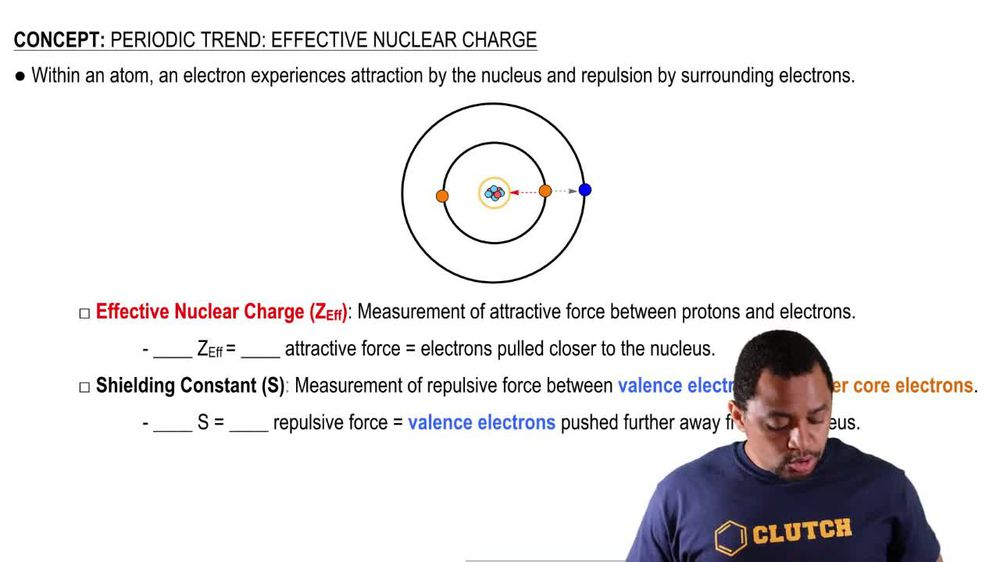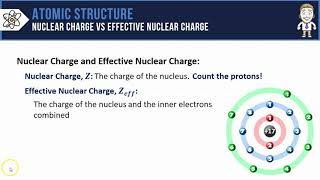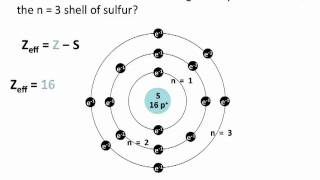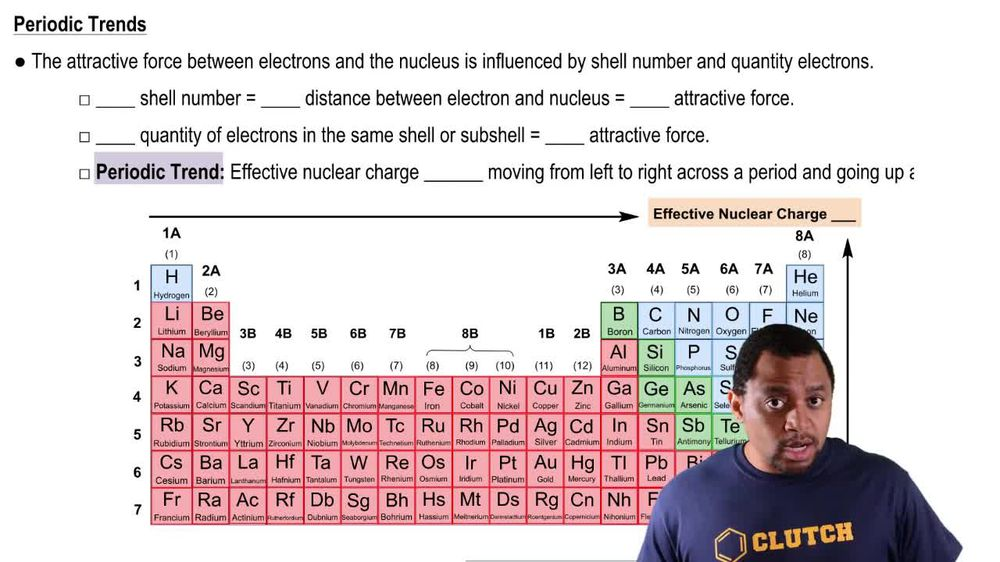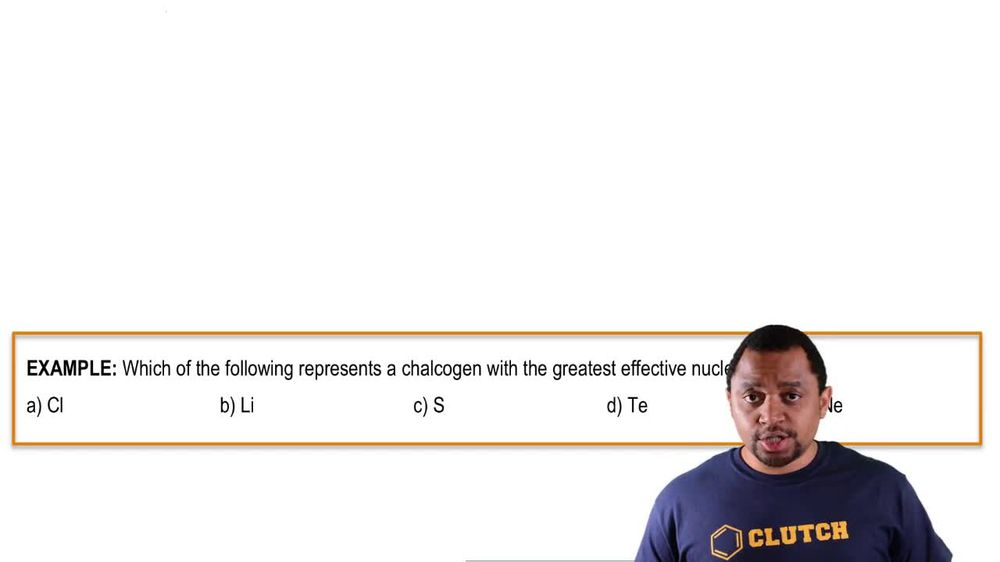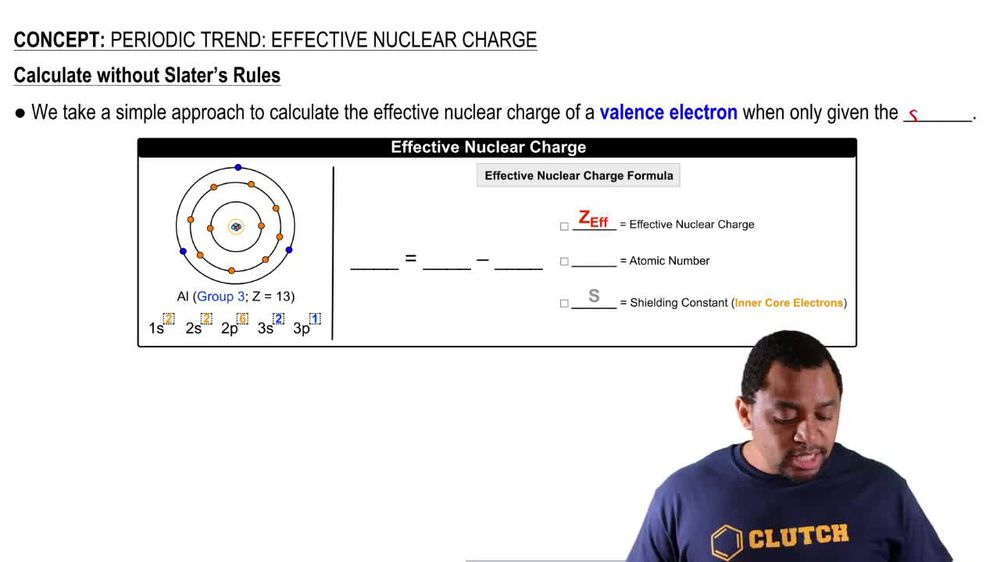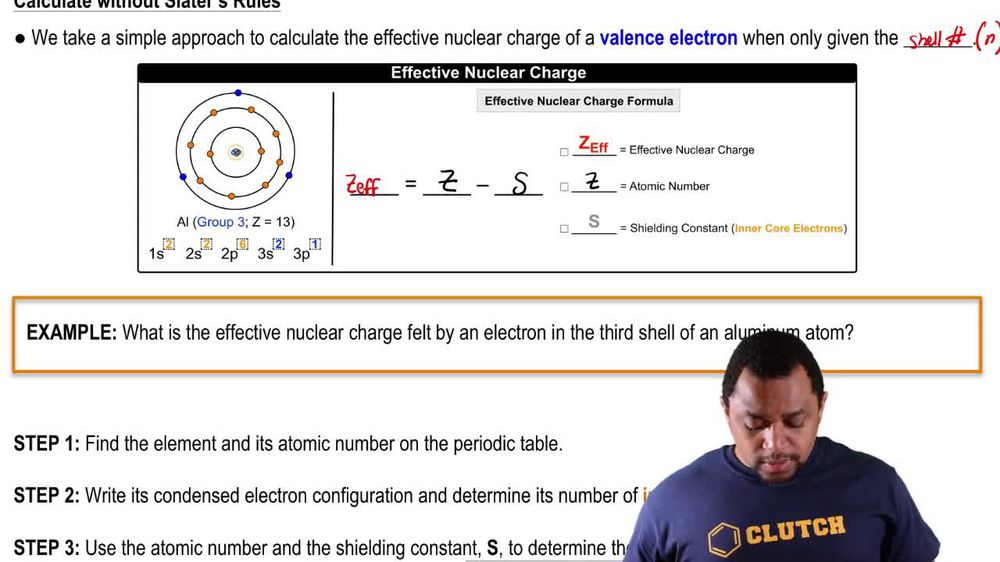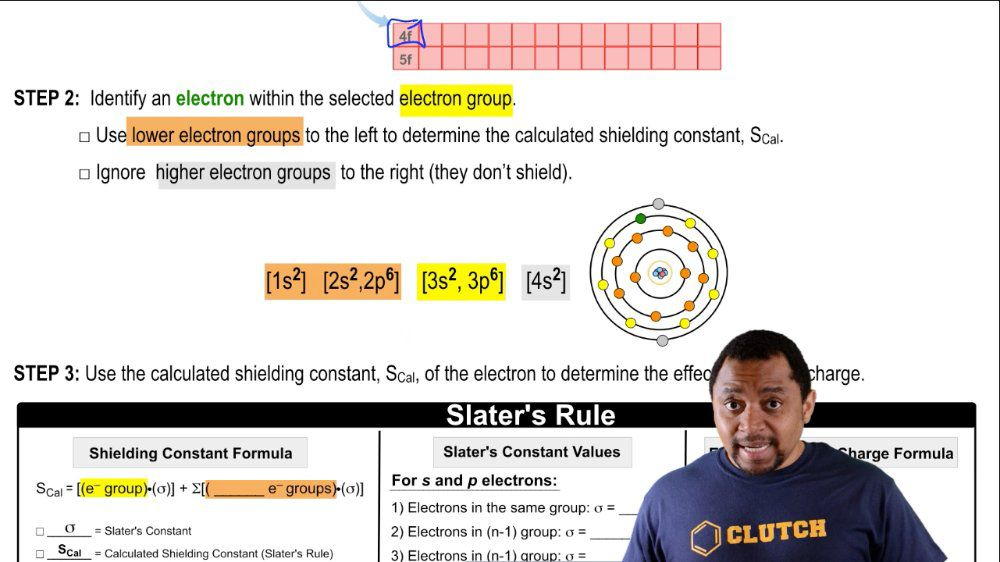Start typing, then use the up and down arrows to select an option from the list.
1. 10. Periodic Properties of the Elements2. Periodic Trend: Effective Nuclear Charge# Periodic Trend: Effective Nuclear Charge Example 2

by Jules Bruno
185 views
0
So here we're gonna answer this example question Based on the image we have above here, it says, what is the effective nuclear charge felt by an electron in the third shell? Often aluminum atom. So the steps we take is we find the element and its atomic number on the periodic table here, we already know that aluminum has an atomic number 13. We're going to write the condensed electron configuration and determine its number of inner core electrons. So if we wanted to do the condensed electron configuration instead of writing all of this, we were just write it as neon, followed by three s 23 p one, we'd say Here, remember, our inner core electrons are just the electrons that are not on the outer shelf. So here we can see that since it's in group three A. It has three valence electrons. It has a total of 13 electrons, though, so you do 13 minus three, and that difference will be the number of inner core electrons. So it has 10 inner core electrons. Now we're gonna use the atomic number and the shielding constant to determine the effect of nuclear charge. So here we say that the effective nuclear charge felt by an electron in the third shell of of aluminum atom equals Z minus s. So that's 13 1st atomic number minus. It's shielding constant, which remember, is equal to the number of inner core electrons. So that's 10. So that equal plus three. That means an electron in the third shell would feel a plus three effective nuclear charge or attractive force from the nucleus. So this is a simplified way of looking at the effect of nuclear charge of any electron within a given Adam.